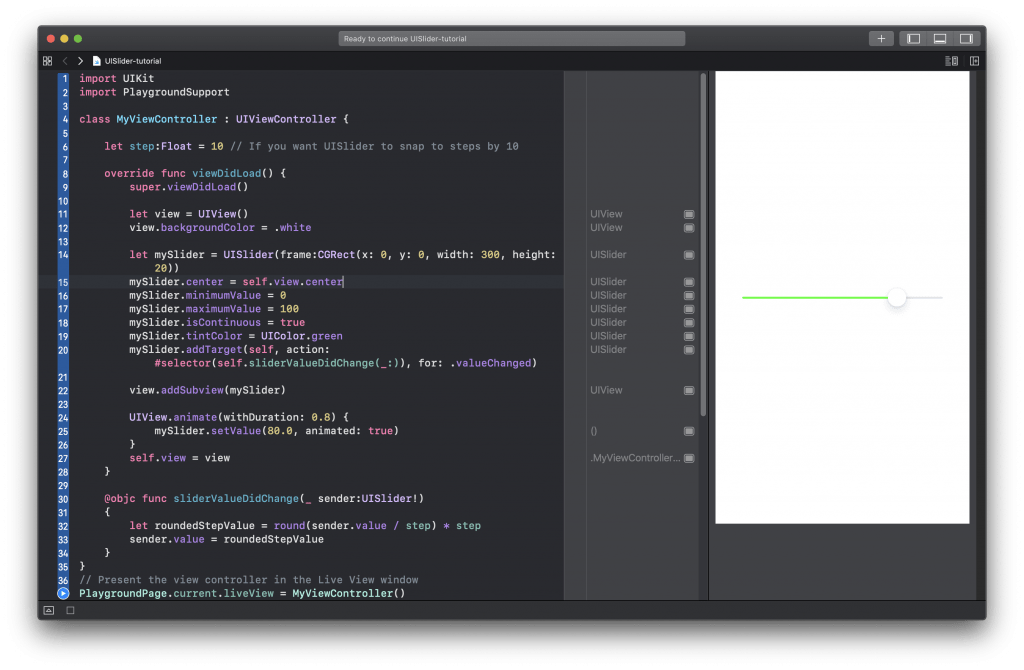# Create UISlider in Swift Programmatically

In this tutorial, you will learn how to create UISlider in Swift programmatically. UISlider is a UI control that is used to select a single value from a continuous range of values. Below is an image of UISlider control.## Create UISlider Programmatically

To create UISlider control programmatically, you need to create a new instance of the UISlider object. Below is a Swift code example that creates a new slider control and positions it in the center of the view.

```let view = UIView()

let mySlider = UISlider(frame:CGRect(x: 0, y: 0, width: 300, height: 20))
mySlider.center = self.view.center

## Configuring UISlider Control

UISlider has many properties that you can configure programmatically. For example, you can set a minimum and a maximum slider value. You can set a slider color, disable or enable continuous scrolling, handle sider events and more. For a complete list of slider control properties check its documentation.

Below is an example of how to access and set a few slider properties.

```mySlider.minimumValue = 0
mySlider.maximumValue = 100
mySlider.isContinuous = true
mySlider.tintColor = UIColor.green```

## Handle Slider Events

You can handle slider events and read slider value as soon as it got changed. To do that you need to add a target function that slider will call when the slider value is changed.

```mySlider.addTarget(self, action: #selector(self.sliderValueDidChange(_:)), for: .valueChanged)
```

If you add a target function to a slider, by default, it will be called continuously as the user slides the slider. To change that behavior and make the function be called only when the slider stops sliding, set the isContinuous property to false.

`mySlider.isContinuous = false`

## Slide Step By Step

By default, slider slides continuously. You can change that behavior and make the slider snap to steps by 5 or 10 points. TO see how it works, try using the below function when slider value changes.

```@objc func sliderValueDidChange(_ sender:UISlider!)
{
print("Slider value changed")

// Use this code below only if you want UISlider to snap to values step by step
let roundedStepValue = round(sender.value / step) * step
sender.value = roundedStepValue

print("Slider step value \(Int(roundedStepValue))")
}```

## Set Initial UISlider Value

You can set initial slider value and if needed animate it. For example, below is a short Swift code snippet that sets initial slider value and creates a little animation to make the slider slowly slide.

``` UIView.animate(withDuration: 0.8) {
mySlider.setValue(80.0, animated: true)
}```

## Complete Code Example

Below is a complete code example that demonstrates how to create UISlider and how to read slider value as it is being used. Try running it in your Xcode project to see how it works.

```import UIKit
import PlaygroundSupport

class MyViewController : UIViewController {

let step:Float = 10 // If you want UISlider to snap to steps by 10

let view = UIView()
view.backgroundColor = .white

let mySlider = UISlider(frame:CGRect(x: 0, y: 0, width: 300, height: 20))
mySlider.center = self.view.center

mySlider.minimumValue = 0
mySlider.maximumValue = 100
mySlider.isContinuous = true
mySlider.tintColor = UIColor.green

UIView.animate(withDuration: 0.8) {
mySlider.setValue(80.0, animated: true)
}

self.view = view
}

@objc func sliderValueDidChange(_ sender:UISlider!)
{
print("Slider value changed")

// Use this code below only if you want UISlider to snap to values step by step
let roundedStepValue = round(sender.value / step) * step
sender.value = roundedStepValue

print("Slider step value \(Int(roundedStepValue))")
}
}
// Present the view controller in the Live View window
PlaygroundPage.current.liveView = MyViewController()
```I hope this Swift tutorial, was helpful to you. Have a look at other Swift tutorial on this website. I think you might find other very useful ones.

Happy Swift coding! 🙋🏻‍♂️